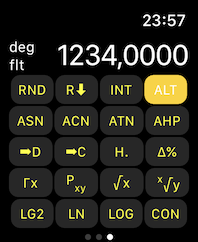π2 is a scientific calculator worn at your wrist by using the Apple Watch. It uses the so called reversed polish notation (RPN) for the input. Multiple mathematical, scientific as well as logical and binary functions for programmers are implemented. Calculations can be done with integer, real or complex numbers.

Besides others these functions are supported:
• calculation of the number of combinations, or permutations using integer numbers
• faculty function for integer numbers and gamma-function for positive real numbers
• trigonometrical, logarithmic, exponential und hyperbolic function for real and complex numbers
• date and time conversions
• logical operationens for binary, octal or hex numbers
• cartesian into polar coordinate conversions and vice versa
• angle transformations
• calculation of absote values (real and complex numbers)
• determination of the integer or fractional part of a real number
• rounding of real numbers
• random number generator

The calculator has a stack depth of ten and supports ten memory registers for all number types. The stack as well as the memory registers can be inspected. The stack can be manipulated by various operations, e.g. the topmost two stack elements can be swapped.